To practice completing the trinomiual square go to the circle worksheet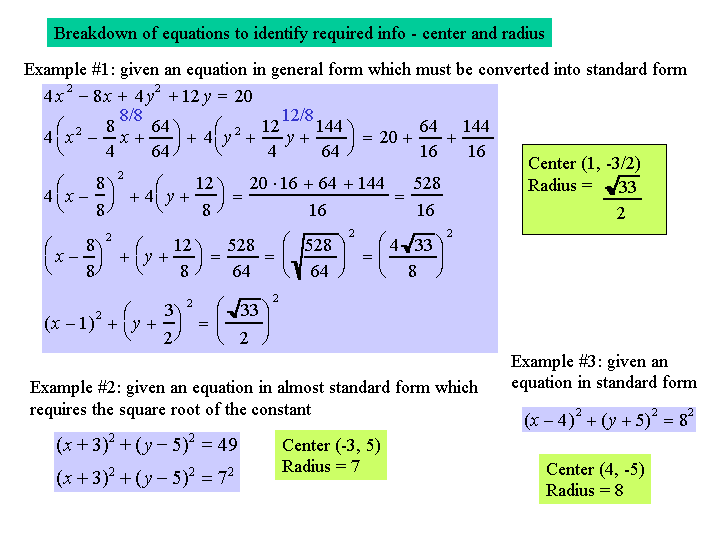To practice completing the trinomiual square and breaking down an equation go to the circle worksheet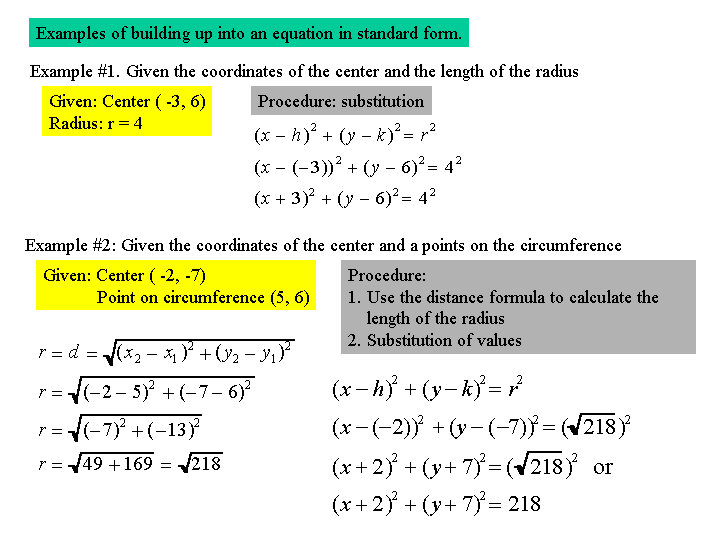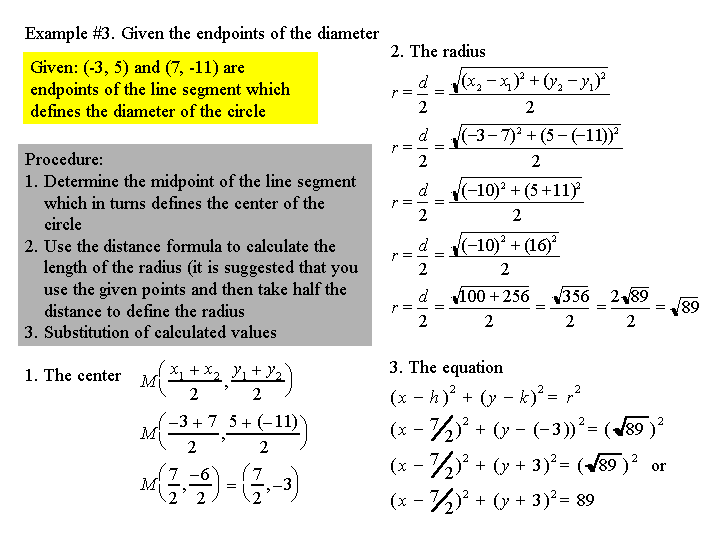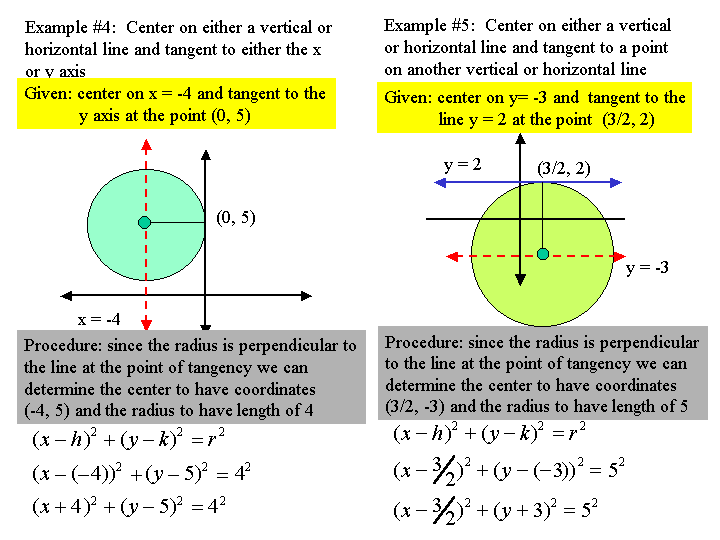To practice building up an equation of a circle go to the circle worksheet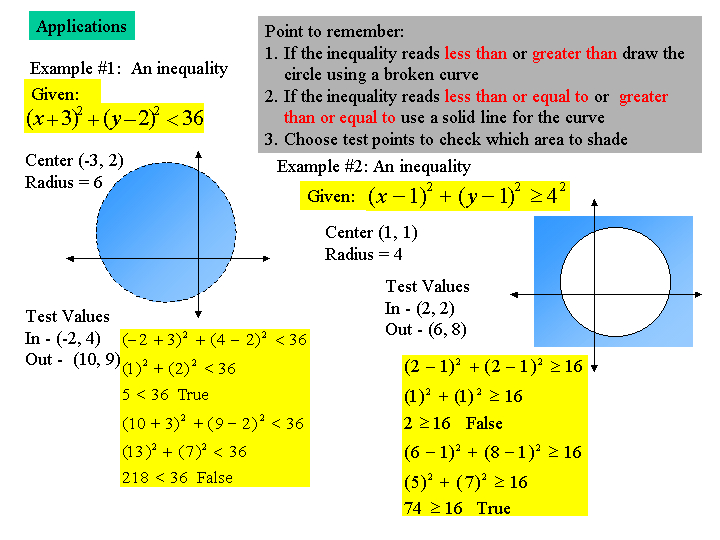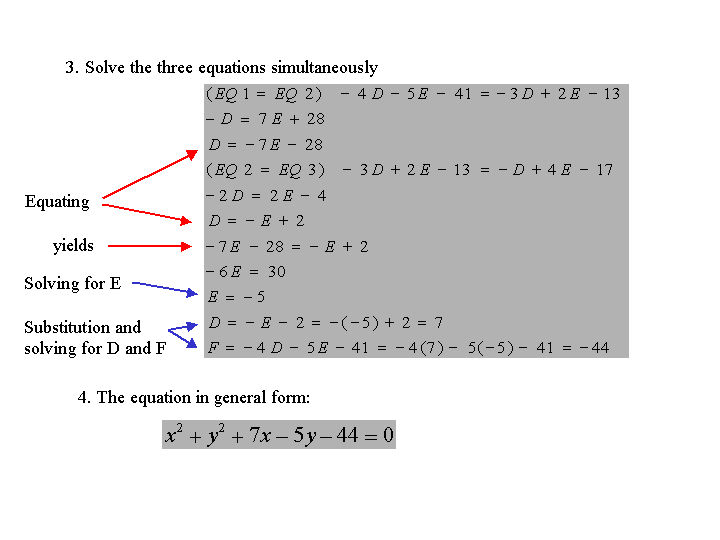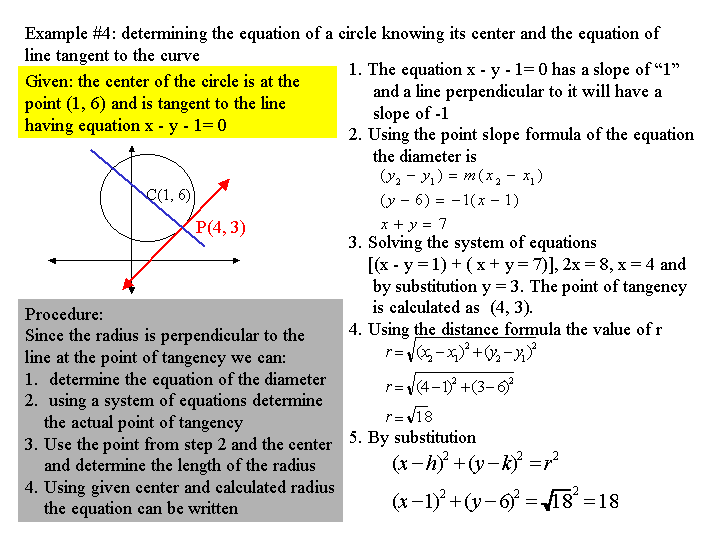To practice a few application questions go to the circle worksheet